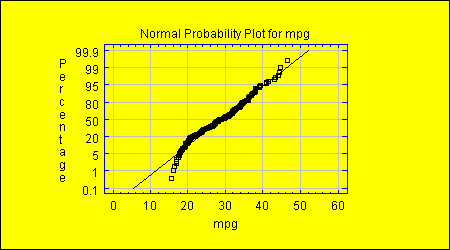# ProbabilityPlot STATBEAN®

Purpose: Creates a probability plot for a single column of numeric data. Allows Statgraphics to function as a probability statistics calculator.

DataSource: Any.Probability Statistics Calculator Read/Write Properties
 Name Type Description Possible Values Default Value distribution String Type of distribution for which plot is constructed. "Exponential","Extreme Value","Logistic","Lognormal","Normal","Uniform","Weibull" "Normal" drawLine boolean Whether to add a line to the plot. true,false true drawVertical boolean Whether to plot the percentages on the vertical axis. true,false true tablewiseExclusion boolean Whether all rows of the data table containing a missing value in any column should be excluded from the plot. true,false false xVariableName String The name of the column with data values to be plotted on the horizontal axis. Any string. ""
Probability Statistics Calculator Code Sample

//create a datasource bean
FileDataSource fileDataSource1 = new STATBEANS.FileDataSource();

//set the file name to be read
fileDataSource1.setFileName("c:\\statbeans\\samples\\spc.txt");

//create a chart bean
ProbabilityPlot probabilityplot1 = new STATBEANS.ProbabilityPlot();

//set the column to be plotted
probabilityplot1.setXVariableName("strength");

//define the plot type
probabilityplot1.setDistribution("Normal");

//show the plot